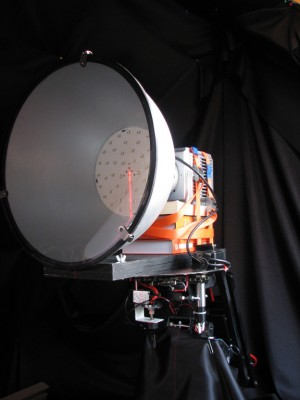# OliNo

Renewable Energy

## Line Lite International BV High Bay Industrial LED Fixture 48 pcs CREE IP40 – 100-240 VACpresents a big light bulb with 48 power leds in it. It was a challenge to measure it on BigMama, but it was managed. This article shows the measurement results. Many parameters are also found in the Eulumdat file.

See this overview for a comparison with other light bulbs.

### Summary measurement data

parameter meas. result remark
Color temperature 7887 K Bright white (or cold white)
Luminous intensity Iv 1667 Cd
Beam angle 64 deg
Power P 54 W
Power Factor 0.70 For every 1 kWh net power consumed, there has been 1.0 kVAhr for reactive power..
Luminous flux 1778 lm
Luminous efficacy 33 lm/W
CRI_Ra 77 Color Rendering Index.
Coordinates chromaticity diagram x=0.2971 and y=0.3030
Fitting 230V
PAR-value 16.9 μMol/s/m2 The number of photons seen by an average plant when it is lit by the light of this light bulb. Value valid at 1 m distance from light bulb.
D x H external dimensions 475 x 600 mm External dimensions of the light bulb.
D luminous area 440 mm Dimensions of the luminous area (used in Eulumdat file). This is equal to the diameter of the reflector’s front side.
form factor pendant
General remarks The ambient temperature during the whole set of measurements was 30.5-32 deg C.Warm up effect: during the warm up time the illuminance decreased with 11 % and the consumed power with 7 %.

Voltage dependency: the power consumption and illuminance do not vary when the voltage is varied from 200 – 250 V.

This article contains additional photos of the light bulb and the way it was mounted on BigMama.

Measurement report (PDF)Eulumdat fileRight click on icon and save the file.

### Overview table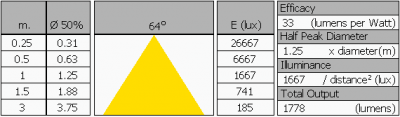Note that the values at 1 m distance are measured and the values at other distances are computed from this value.

### Eulumdat light diagram

An interesting graph is the light diagram, indicating the intensity in the C0-C180 and the C90-C270 plane. This light diagram below comes from the program Qlumedit, that extracts these diagrams from an Eulumdat file.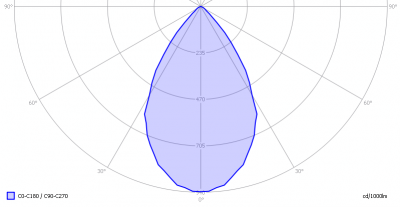The light diagram giving the radiation pattern.

It indicates the luminous intensity around the light bulb. This light bulb has the same light diagram for the C0-C180 as for the C90-C270 plane since it has symmetry over its height axis.

The unit is Cd/1000lm, meaning the intensity in Cd assuming there would be 1000 lumen in the measured light bulb. This enables comparing different types of light bulbs.

### Illuminance Ev at 1 m distance, or luminous intensity Iv

Herewith the plot of the averaged luminous intensity Iv as a function of the inclination angle with the light bulb.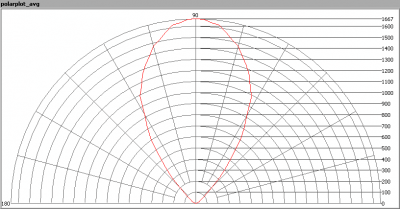The radiation pattern of the light bulb.

This radiation pattern is the average of the light output of the light diagram given earlier. Also, in this graph the luminous intensity is given in Cd.

These averaged values are used (later) to compute the lumen output.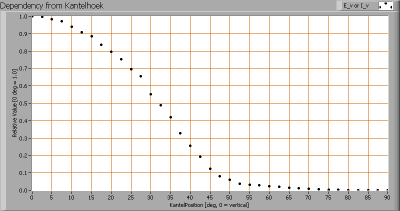Intensity data of every measured turn angle at each inclination angle.

This plot shows per inclination angle the intensity measurement results for each turn angle at that inclination angle. There normally are differences in illuminance values for different turn angles. However for further calculations the averaged values will be used.

When using the average values per inclination angle, the beam angle can be computed, being 64 degrees. This is the average value but as the light bulb has a symmetry over its z-axis, this angle is valid for all turn-angles the light bulb is looked at.

### Luminous flux

With the averaged illuminance data at 1 m distance, taken from the graph showing the averaged radiation pattern, it is possible to compute the luminous flux.

The result of this computation for this light spot is a luminous flux of 1778 lm.

### Luminous efficacy

The luminous flux being 1778 lm, and the power of the lightbulb being 54 W, yields a luminous efficacy of 37 lm/W.

A power factor of 0.70 means that for every 1 kWh net power consumed, a reactive component of 1.0 kVAr was needed.

 Light bulb voltage (used on power supply!) 230 VAC Light bulb current 338 mA Power P 54 W Apparent power S 78 VA Power factor 0.70

Of this light bulb the voltage across ad the resulting current through it+its constant current driver power supply are measured and graphed.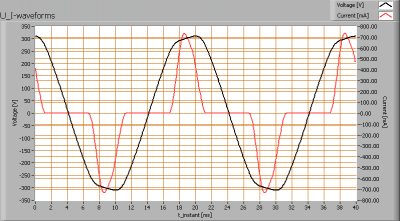Voltage across and current through the lightbulb

The current is different from 0 and peaks when the voltage is close to its maximum value. The current’s peak is somewhat symmetrical so it is in phase with the voltage. Due to the mismatch in form factors the power factor is less than 1.

Also the power spectrum of the current is determined.Current power spectrum in % of the first harmonic (50 Hz).

The current peaks result in a current power spectrum with some higher harmonics.

### Color temperature and Spectral power distribution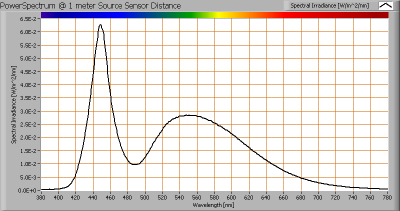The spectral power distribution of this light bulb, energies on y-axis valid at 1 m distance.

The measured color temperature is about 7900 K which is cold white.

This color temperature is measured straight underneath the light bulb. Below a graph showing the color temperature for different inclination angles.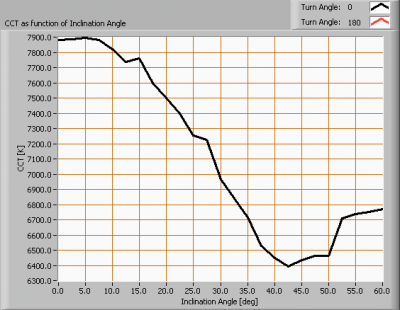Color temperature as a function of inclination angle.

The color temperature is given for inclination angles up to 60 degrees, since at higher angles there is almost no light output anymore.

The value decreases with 19%.

### PAR value and PAR spectrum

To make a statement how well the light of this light bulb is for growing plants, the PAR-area needs to be determined. PAR stands for Photosynthetic Active Radiation which is that radiation that participates actively to photosynthesis and is expressed in μMol/s/m2.

Photosynthesis is the essence for growth and flowering of plants, where the blue part of the light spectrum is responsible for growth and the red part is responsible for flowering and budding (for gemmation). For photosynthesis it is more important to count the number of photons rather than the power in the light.

The power spectrum (power per wave length) of the light of the light bulb is converted into the number of photons (number of photons per wave length) and then these number of photons is weighted against the relative sensitivity of an average plant (which varies in value dependent on the wave length). The relative sensitivity curve in DIN-norm 5031-10:2000 is used for this. The next image shows the result.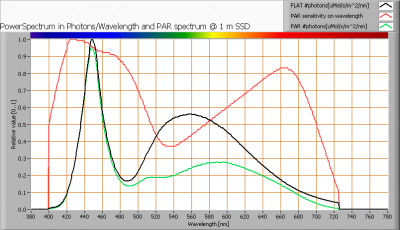The photon spectrum, then the sensitivity curve and as result the final PAR spectrum of the light of this light bulb

The black curve gives the power spectrum of the light of this light bulb, in number of photons per wave length.

In red the relative sensitivity curve of an average plant (according to DIN norm 5031-10:2000).

Multiplying these two results in a curve showing the number of photos per wave length of the light of this light bulb, the PAR spectrum.

Summing all the photons, gives a PAR number that for this light results in 16.9 μMol/s/m2. This value is valid at 1 m distance from the light bulb.

Dividing the PAR number by the total number of photons of the light of this light bulb gives a sort of PAR efficiency, which is 67 % (valid for the PAR wave length range of 400 – 725 nm). So maximally 67 % of the total of photons in the light is effectively used by the average plant (since the plant might not take 100 % of the photons at the frequency where its relative sensitivity is 100 %).

Note: when mentioning this percentage of efficiency, it is important to verify if all wavelengths are present in a sufficient manner. Not that only blue light is available, when the plant is going to be used for flowering where specifically red light is needed.

### Chromaticity diagram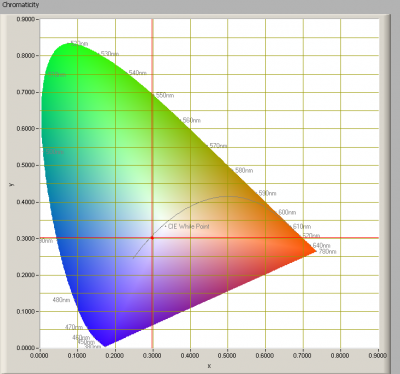The chromaticity space and the position of the lamp’s color coordinates in it.

The light coming from this lamp is close to the Planckian Locus (the black path in the graph).

Its coordinates are x=0.2971 and y=0.3030.

### Color Rendering Index (CRI) or also Ra

Herewith the image showing the CRI as well as how well different colors are represented (rendered). The higher the number, the better the resemblance with the color when a black body radiator would have been used (the sun, or an incandescent lamp).

Each color has an index Rx, and the first 8 indexes (R1 .. R8) are averaged to compute the Ra which is equivalent to the CRI.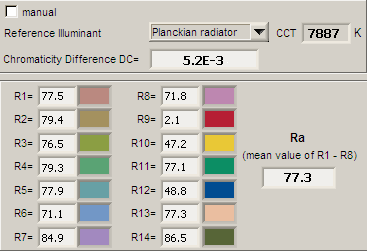CRI of the light of this lightbulb.

The value of 77 is lower than 80 which is considered a minimum value for indoor usage.

Note: the chromaticity difference is 0.0052 indicates the distance to the Planckian Locus. Its value is lower than 0.0054, which means that the calculated CRI result is meaningful.

### Voltage dependency

The dependency of a number of lamp parameters on the lamp voltage is determined. For this, the lamp voltage has been varied and its effect on the following light bulb parameters measured: illuminance E_v [lx], the lamppower P [W], the (Correlated) Color Temperature [K] and the luminous efficacy [lm/W].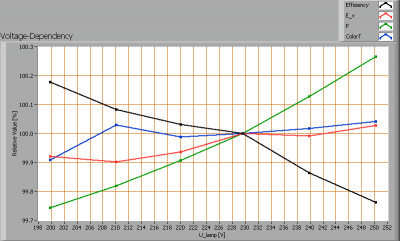Lamp voltage dependencies of certain light bulb parameters, where the value at 230 V is taken as 100 %.

The consumed power and illuminance vary < 0.3 % when the voltage varies between 200-250 V. This variation is insignificant.

When the voltage at 230 V varies with + and – 5 V, then the illuminance varies within 0.1 %, so when abrupt voltage changes occur this effect is not visible in the illuminance output.

### Warm up effects

After switch on of a cold lamp, the effect of heating up of the lamp is measured on illuminance E_v [lx], color temperature CT or correlated color temperature CCT [K], the lamppower P [W] and the luminous efficacy [lm/W].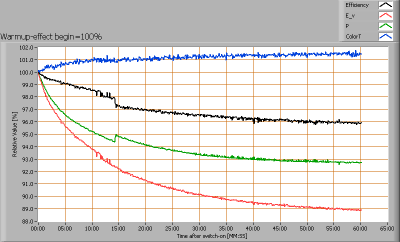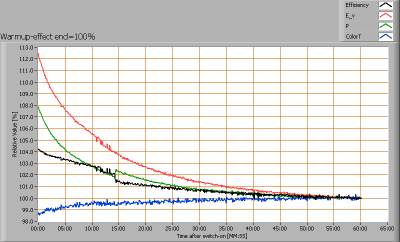Effect of warming up on different light bulb parameters. At top the 100 % level is put at begin, and at bottom at the end.

The warm-up time is about 1 hour, during which the illumination drops 11 % and the consumed power drops about 7 %.